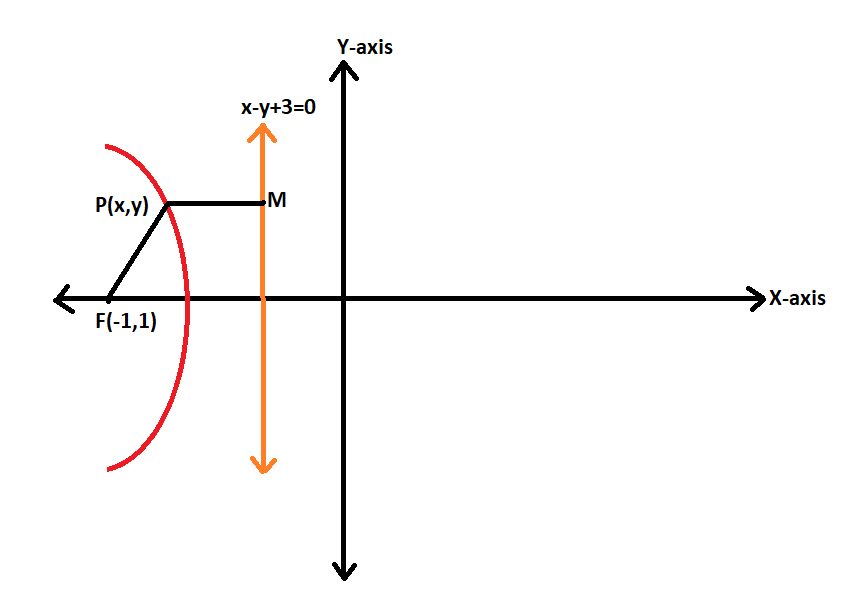Courses
Courses for Kids
Free study material
Offline Centres
MoreLast updated date: 29th Nov 2023
Total views: 280.8k
Views today: 5.80k

# If the equation of a directrix of a hyperbola is $x - y + 3 = 0$. Its focus is $\left( { - 1,1} \right)$ and the eccentricity is 3. Find the equation of the hyperbola.Verified
280.8k+ views
Hint: To get the equation of the hyperbola we will consider a point P on the hyperbola. Then there is given the equation of the directrix and the position of focus. We will find two equations. One if from P to directrix and other is from focus to the point P. equating them will give the equation of hyperbola.

Complete step by step solution:
Given the equation of the directrix as $x - y + 3 = 0$.
And the focus is $\left( { - 1,1} \right)$
Now let P(h,k) be the point on the hyperbola. Now let’s draw the situation.Now we know that the P is the point on the hyperbola.
We know that,
$FP = e \times PM$
Eccentricity is given to us. Then we will find the distance FP using the distance formula.
Let P(h,k) and F(-1,1) be the other point.
$FP = \sqrt {{{\left( {{x_2} - {x_1}} \right)}^2} + {{\left( {{y_2} - {y_1}} \right)}^2}}$
Putting the values we get,
$FP = \sqrt {{{\left( {h - \left( { - 1} \right)} \right)}^2} + {{\left( {k - 1} \right)}^2}}$
$FP = \sqrt {{{\left( {h + 1} \right)}^2} + {{\left( {k - 1} \right)}^2}}$
Now the PM is perpendicular to the directrix and we know that the length of that perpendicular is given by,
$PM = \left| {\dfrac{{a{x_1} + b{y_1} + c}}{{\sqrt {{a^2} + {b^2}} }}} \right|$
Now the equation of the line is nothing but the directrix. Thus comparing it with the equation of directrix $x - y + 3 = 0$ and the point is P(h,k)
Then the above length will be,
$\left| {\dfrac{{h - k + 3}}{{\sqrt 2 }}} \right|$
But we know that,
$FP = e \times PM$
$\sqrt {{{\left( {h + 1} \right)}^2} + {{\left( {k - 1} \right)}^2}} = 3\left| {\dfrac{{h - k + 3}}{{\sqrt 2 }}} \right|$
Taking the square on both sides,
${\left( {h + 1} \right)^2} + {\left( {k - 1} \right)^2} = 9 \times \left[ {\dfrac{{\left( {h - k + 3} \right)\left( {h - k + 3} \right)}}{2}} \right]$
Taking the square,
${h^2} + 2h + 1 + {k^2} - 2k + 1 = 9 \times \left[ {\dfrac{{h\left( {h - k + 3} \right) - k\left( {h - k + 3} \right) + 3\left( {h - k + 3} \right)}}{2}} \right]$
Now multiplying the terms on RHS,
${h^2} + 2h + {k^2} - 2k + 2 = 9 \times \left[ {\dfrac{{{h^2} - hk + 3h - kh + {k^2} - 3k + 3h - 3k + 9}}{2}} \right]$
${h^2} + 2h + {k^2} - 2k + 2 = 9 \times \left[ {\dfrac{{{h^2} + {k^2} - 2hk + 6h - 6k + 9}}{2}} \right]$
On taking the 2 on LHS,
$2\left( {{h^2} + 2h + {k^2} - 2k + 2} \right) = 9\left( {{h^2} + {k^2} - 2hk + 6h - 6k + 9} \right)$
Now multiplying the numbers insides the bracket,
$9{h^2} - 2{h^2} + 9{k^2} - 2{k^2} - 18hk + 54h - 4h - 54k + 4k + 81 - 4 = 0$
Now taking the common coefficient terms and performing the operation,
$7{h^2} + 7{k^2} - 18hk + 50h - 50k + 77 = 0$
Now replacing the h and k terms by x and y we will get the equation of the hyperbola.
$7{x^2} + 7{y^2} - 18xy + 50x - 50y + 77 = 0$
This is the equation of the hyperbola.

Note:
Note that the way we find the equation is using the simple formulas. Such that the equation of the directrix and the focus is the help to find the equation. Also note that when we find the length of distance from the point on hyperbola to directrix we used one more formula. For that P is the point and directrix is the line.